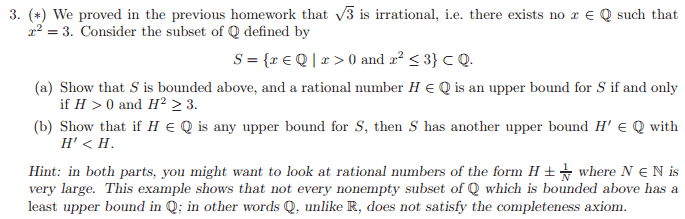# Introductory analysis question

synkkI've split the proof for part a) into 3 parts:

here's what I have thus far:

1) to prove H is an upper bound consider: $H < 0$ as an upper bound for S. We take $H = -1$ and hence $H^2 = 1$ and $1 \leq 3$. 1 is in the set S and hence $H < 0$is not an upper bound which implies that $H > 0$ is an upper bound.

2) consider $H^2 < 3$is an upper bound, and for N belonging to the natural numbers $H + 1/N$ is a rational number. Consider $(H+1/N)^2 = H^2 + 2H/N + 1/N^2 < 3$for N sufficiently large. This implies that $H + 1/N$ is in the set S, but$H + 1/N > H$ hence a contradiction therefore$H^2 < 3$ is not an upper bound and $H^2 \geq 3$ is.

3)if H is a rational number and $H >0$, $H^2 \geq3$ we see $H\geq \sqrt{3}$ as $H > 0$ thus all $H\geq \sqrt{3}$ are upper bounds as $x \leq \sqrt{3}$. That is all I have for part a),

is this correct?

for part b) here is what I have:
I called $H' = H - 1/N$ and tried to prove it by contradiction, i.e. assume$H - 1/N <\sqrt{3}$ but I got to $H < \sqrt{3} + 1/N$ and we know $H \geq \sqrt{3}$ from part a), and for N sufficiently large 1/N = 0, but I don't really think this is a good enough contradiction, for instance. $\sqrt{3}\leq H < \sqrt{3} + 1/N$ is ok, if N is say 2 or so. How would I go about contradicting this?

Staff Emeritus
Gold Member
2021 Award
Part 1 is not good, as all you've shown is that -1 would be in the set if negative numbers were allowed in the set (which they aren't anyway).

Part 2 and 3 are basically OK but you have some language issues (which also show up in part 1). When you say
therefore $H^2 < 3$ is not an upper bound and $H^2 \geq 3$ is
you haven't said anything meaningful. $H^2 \geq 3$ isn't an upper bound, it's a condition which upper bounds must satisfy. A correct statement would be something like 'therefore if H is an upper bound, $H^2 \geq 3$.

For part b you basically want to copy your (2) idea. You know that if H is an upper bound of S in Q then H is not equal to $\sqrt{3}$ - or you should go ahead and prove that the square root of 3 is irrational - so H2 > 3 strictly. Therefore (H-1/N)2 > 3 if N is small enough (you have to prove this).

synkk
Part 1 is not good, as all you've shown is that -1 would be in the set if negative numbers were allowed in the set (which they aren't anyway).

Part 2 and 3 are basically OK but you have some language issues (which also show up in part 1). When you say

you haven't said anything meaningful. $H^2 \geq 3$ isn't an upper bound, it's a condition which upper bounds must satisfy. A correct statement would be something like 'therefore if H is an upper bound, $H^2 \geq 3$.

For part b you basically want to copy your (2) idea. You know that if H is an upper bound of S in Q then H is not equal to $\sqrt{3}$ - or you should go ahead and prove that the square root of 3 is irrational - so H2 > 3 strictly. Therefore (H-1/N)2 > 3 if N is small enough (you have to prove this).
Thank you for correcting my language issues, I understand my presentation is not very good, but I'm trying to improve.

I don't understand what you mean by (1)? I'm trying to show that if H is an upper bound, then H>0 and H^2 > 3, with 1), I'm using contradiction by assuming H<=0 is the upper bound then going on to show that this is impossible hence H>0 must be an upper bound. Then from 2) I use a similar argument to show H^2 >= 3 must be an upper bound (by contradiction). Could you explain where I've gone wrong here?

synkk
OK for part b) here is what I got so far

Assume that ## (H-1/N)^2 < 3 ## then we have ## H < \sqrt{3} + 1/N ## for ## N \in \mathbb{N} ## as ## H > \sqrt{3} ## we get ## \sqrt{3} < H < \sqrt{3} + 1/N ## and for N very large (specifically for ## N > \frac{1}{H-\sqrt{3}} ## ) we get ## 1/N ## tending to 0, hence ## \sqrt{3} < H < \sqrt{3} ## which is a contradiction hence ## (H-1/N)^2 > 3##

I'm not sure if this is correct, but that's all I can get so far from it, please advise.

DarthRoni
I haven't looked at a problem like this in a while. I came up with this idea.
Take ##P,H\in \mathbb{Q}## Such that ##P>\sqrt{3}>H ## and assume
$$H + \frac{1}{N} \notin (H, P) \forall\ N\in\mathbb{N}\implies H = P = \sqrt{3}$$
since for all rational numbers, take ##x,y \in \mathbb{N}##
$$\frac{x}{y} + \frac{1}{2y} < \frac{x+1}{y}$$
or
$$\frac{x}{y} + (\frac{1}{2})(\frac{1}{y}) < \frac{x}{y} + \frac{1}{y}$$
I believe this proves both a and b

Last edited:
DarthRoni
Hmm I'm not sure if this works but I believe my proof is incomplete.
Assume ##P## is the smallest rational number greater than ##\sqrt{3}##
$$\implies \exists Z\in\mathbb{Q}\ and\ Z\in [H,P]\ |\ Z < \sqrt{3} \implies 3 > Z^2 > H^2$$
I believe we can make the assumption the other way in order to prove that there is an infinite amount of rational numbers across ##[H,P]##
I'm not sure if this is allowed, any feedback would be great !